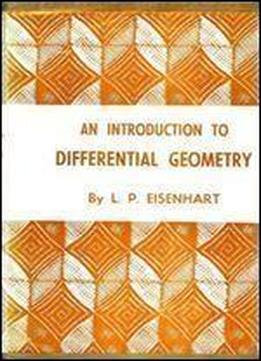# An Introduction To Differential Geometry, With Use Of The Tensor Calculus, by Luther Pfahler Eisenhart / 1940 / English / PDF

This book covers the general equations found in differential Geometry using summation convention as well as Riemann's curvature tensor which is the conditions of integrability of a system of total differential equations with coefficients that are linear in the dependent variables it also covers curvature and torsion Fernet-Serret formulas in terms of general coordinates, geodesic torsion, geodesic curvature, normal curvature and developable surfaces and more.

views: 485